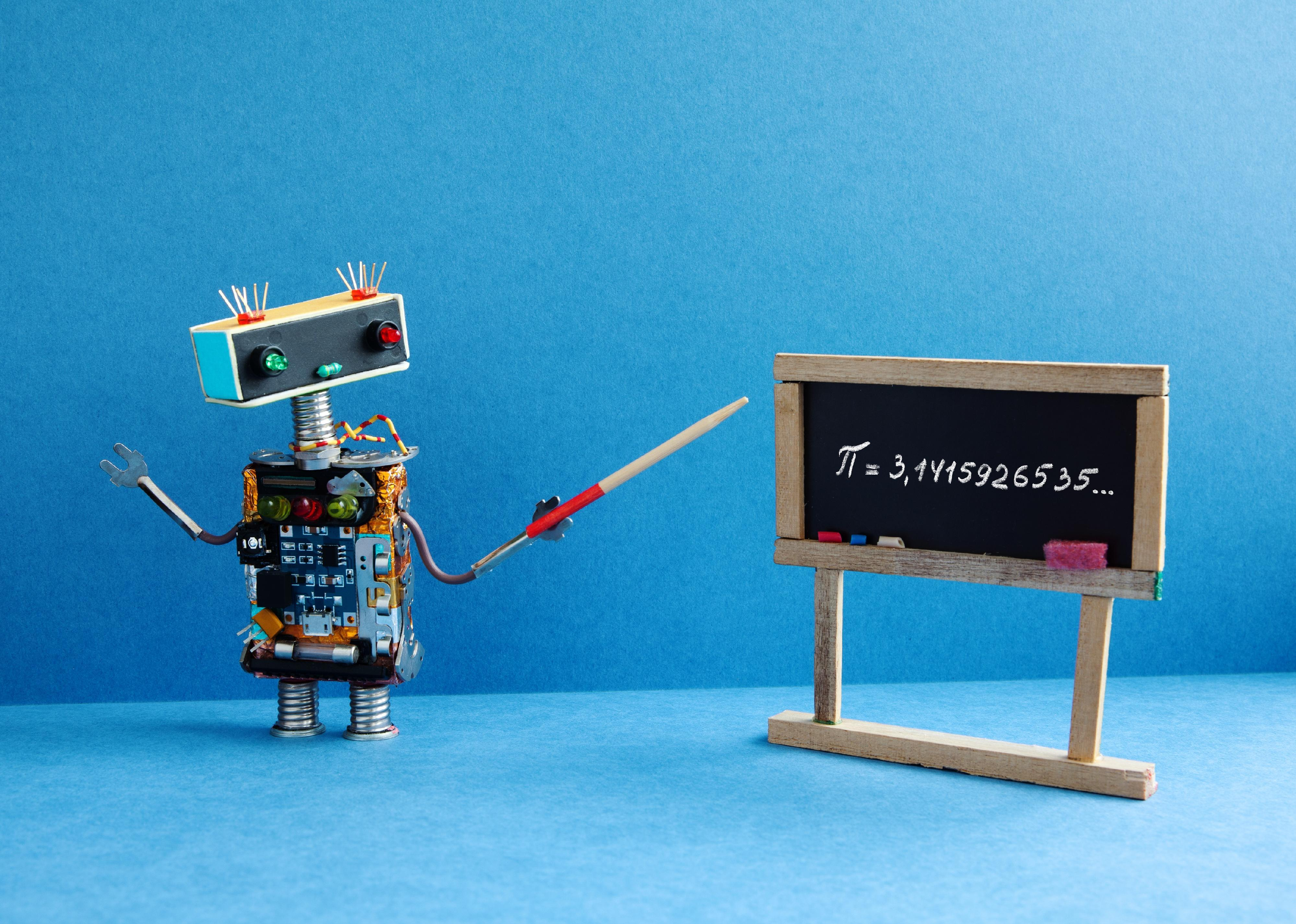# Do you know these 50 math terms?

Written by:
February 13, 2020
Besjunior // Shutterstock

## Do you know these 50 math terms?

Math is defined as "the science of numbers and their operations, interrelations, combinations, generalizations, and abstractions and of space configurations and their structure, measurement, transformations, and generalizations."

While math has baffled and terrified generations of schoolchildren who struggled to wrap their heads around its complexities, those who love math find magic in its secrets. Math is everywhere—in every bridge or building ever constructed, every army ever organized, every medicine ever discovered. Without math, there would be no money, no commerce, no cars, no computers, no science, no music.

Math is as old as humanity. There's evidence that early humans etched notches in bones as primitive forms of tabulation. Basic arithmetic and geometry laid the foundations for algebra and later, for advanced calculus. Math was fundamental to the structures and societies of all the great civilizations, including the early Babylonian, Greek, Egyptian, Chinese, Mesopotamian, Roman, Myan, Indian, and Islamic empires. With each great leap forward in technology, exploration, and science came advancements in mathematics—and like everything else, math has its own language. To do the math, you have to understand the words that represent the concepts.

Everyone knows that to add is to find the sum of numbers and that to subtract is to take away. People of a certain age remember being drilled on times tables and learning for the first time the strange new shapes and images associated with fractions and long division. Those, however, are just the building blocks. Advanced and even intermediate mathematics are described in a vocabulary that's sacred to mathletes but alien to outsiders.

Stacker has compiled a list of key math terms from a variety of authoritative math communication sources, including 2020 data from the Khan Academy, Math Open Reference, and Wolfram Alpha. Although volumes could be and have been written about the concepts they represent, the basic definitions and applications are described below.

You may also like: These baby names are going extinct1 / 50
dreamnikon // Shutterstock

## Distribution

Distribution is an algebraic term that defines the act of spreading terms out equally. Terms are variables or numbers joined by division and/or multiplication. They're distributed by multiplying terms inside of parenthesis with terms outside of the parenthesis.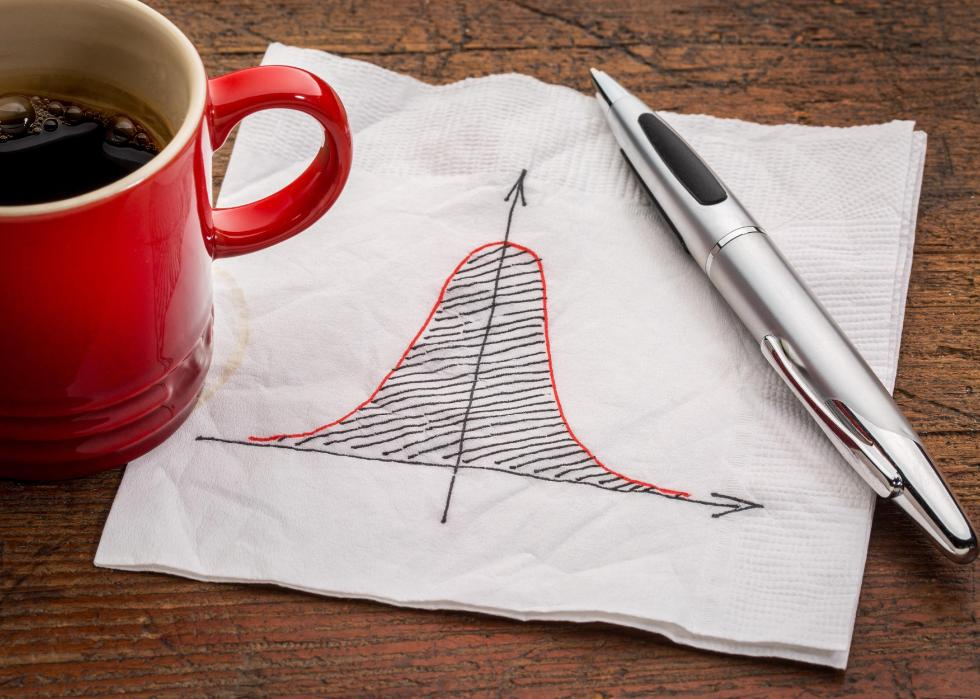2 / 50
marekuliasz // Shutterstock

## Bell curve

A bell curve is a graph that reveals when data are evenly distributed. Bell curves show a small percentage of points on each of the graph's two tails and a larger percentage in the middle.3 / 50
rawf8 // Shutterstock

## Complementary angles

Two angles are complementary when they combine to form a right angle. A 50-degree angle and a 40-degree angle, for example, are complementary because they add up to 90 degrees.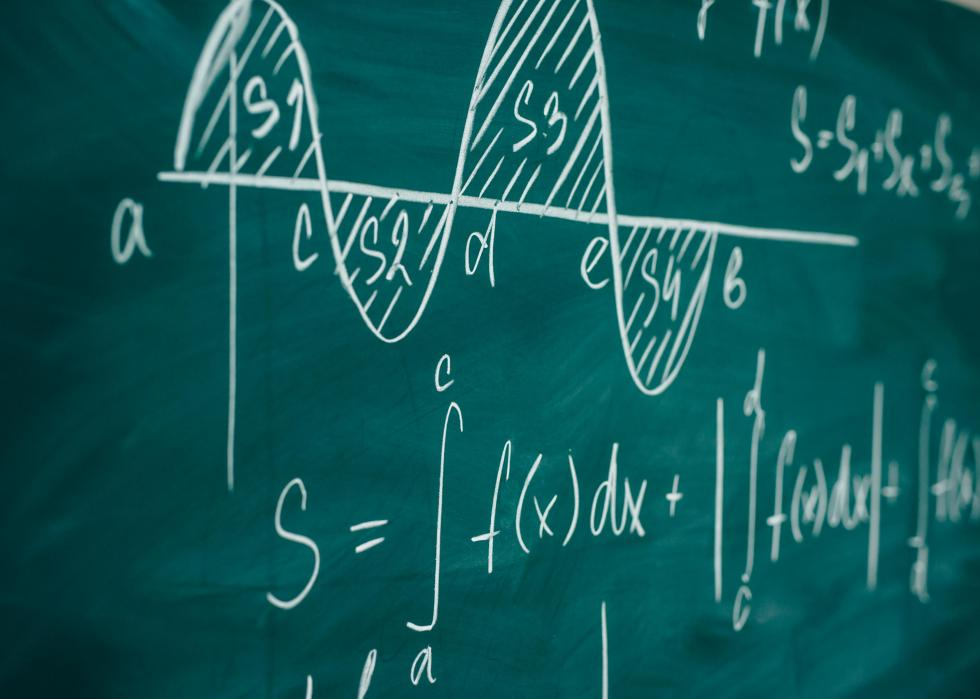4 / 50
Undrey // Shutterstock

## Calculus

Isaac Newton and Gottfried Wilhelm Leibniz are credited with independently developing the field of calculus in the 17th century. There are two branches: Integral calculus determines whole factors through the summation of infinitely numerous smaller factors, and differential calculus is the calculation of rates of change.5 / 50
Things // Shutterstock

## Derivative

Derivatives are models that are used to show rates of change. They can be geometrical, like the slope of a curve, or physical models, which are drawn out in mathematical terms comprising numbers, letters, and symbols.

You may also like: 10 toxic cleaning products and their natural alternatives6 / 50
Iridica // Shutterstock

## Integral

Along with derivatives, integrals are the fundamental objects of calculus. A shortcut method of adding slices to determine a whole, integrals can be used to find many central points, volumes, and areas.7 / 50
Patimat05 // Shutterstock

## Coefficient

A coefficient is a number—the constant—in a variable term in a mathematical expression. The University of Chicago gives the example of 3c + 8d, in which 3 and 8 are coefficients.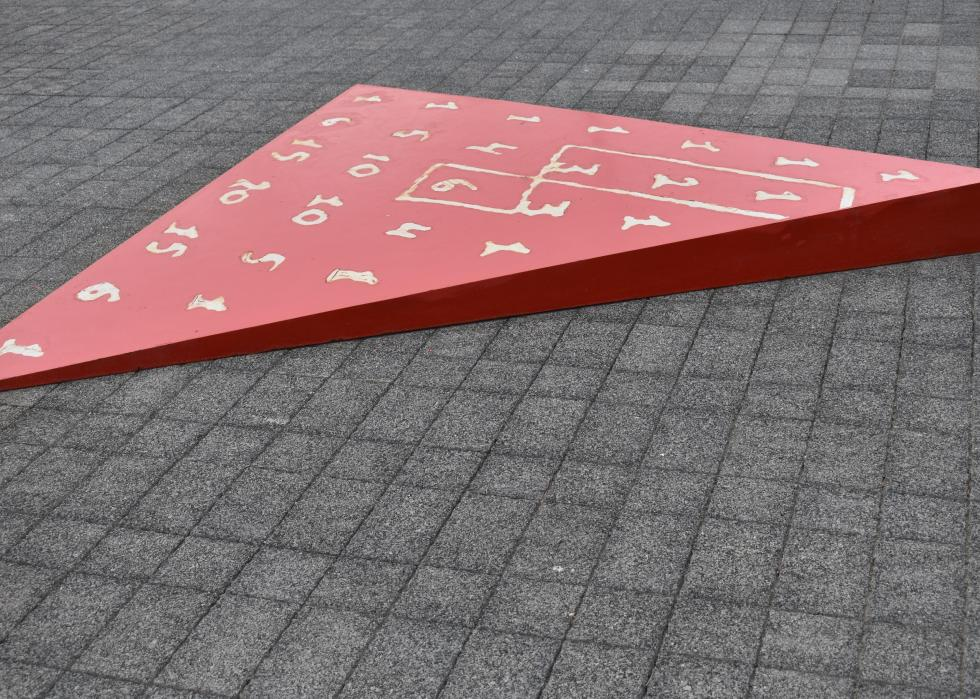8 / 50
alri // Shutterstock

## Pascal's triangle

Pascal's triangle is a visual representation of coefficients arranged in a triangular pattern. It's named for French mathematician Blaise Pascal, but it was used and described by mathematicians in Persia, China, India, Italy, and Germany centuries before.9 / 50

## Conic section

Conic sections are nondegenerate curves that are formed when a cone meets a plane. They can take the form of hyperbola, ellipses, parabolas, and circles.10 / 50
chalermphon_tiam // Shutterstock

## Factor

Every mathematical product contains two or more numbers. Those numbers are called factors.

You may also like: Could you pass the U.S. citizenship test?11 / 50
Lorna Roberts // Shutterstock

## Fibonacci sequence

Named after an early Italian mathematician, the Fibonacci sequence is a string of numbers where each number in the sequence is the sum of the two preceding numbers. For example, 0, 1, 1, 2, 3, 5, 8, 13, 21.12 / 50

## Infinite

The concept of infinity can apply to metaphysical, physical, and mathematical concepts that represent something endless or without bounds. In math, a continuous numerical sequence (1, 2, 3, 4, 5, 6…) could be infinite, as could the number of points on a line.13 / 50
T.Dallas // Shutterstock

## Frequency distribution

A frequency distribution is a statistical graph, table, or list that outlines the frequency of various events in a given sample. It's a generally simple model that displays how often something occurs.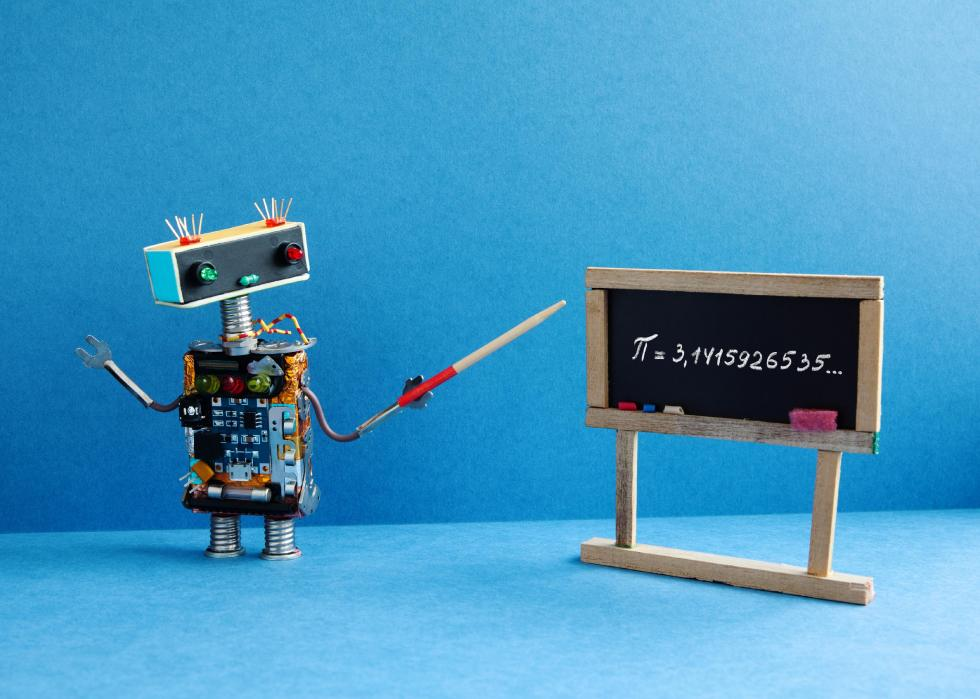14 / 50
Besjunior // Shutterstock

## Irrational numbers

Irrational numbers are real numbers that can't be made by dividing two integers, which have no fractional parts. The decimals of irrational numbers go on infinitely. The most famous irrational number of all is pi.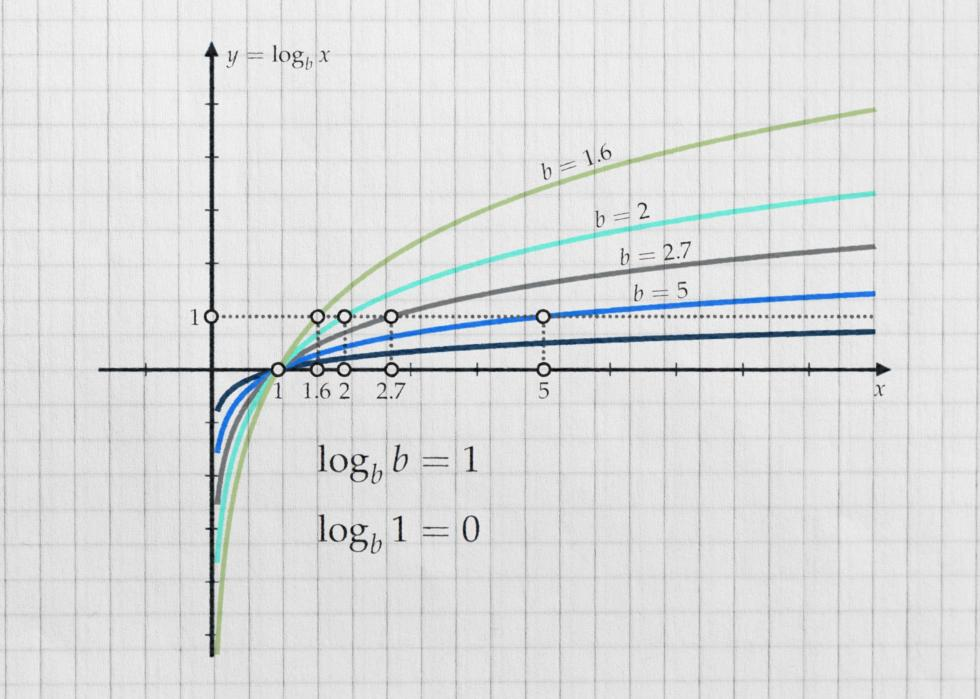15 / 50
benjaminec // Shutterstock

## Logarithm

Mathematicians used logarithms to determine the power to which a base or fixed number has to be raised to get to a given number. Math is Fun defines it simply as "How many of this number do we multiply to get that number?"

You may also like: Scientific breakthroughs from the year you were born16 / 50
Rafael Croonen // Shutterstock

## Prime numbers

Prime numbers, or prime integers, are whole numbers greater than 1 that can't be achieved by multiplying any two other whole numbers other than 1 and itself. The first five prime numbers are 2, 3, 5, 7, and 11.17 / 50
anaken2012 // Shutterstock

## Quartile

Quartiles divide lists of numbers into quarters. When a list is divided into four equal parts, the equal parts become quarterlies.18 / 50
Jacob L. // Shutterstock

## Slope

A slope represents the steepness of a line. Often defined as "rise over run," slopes, and understanding how to determine them, is one of the keys to reading mathematical graphs.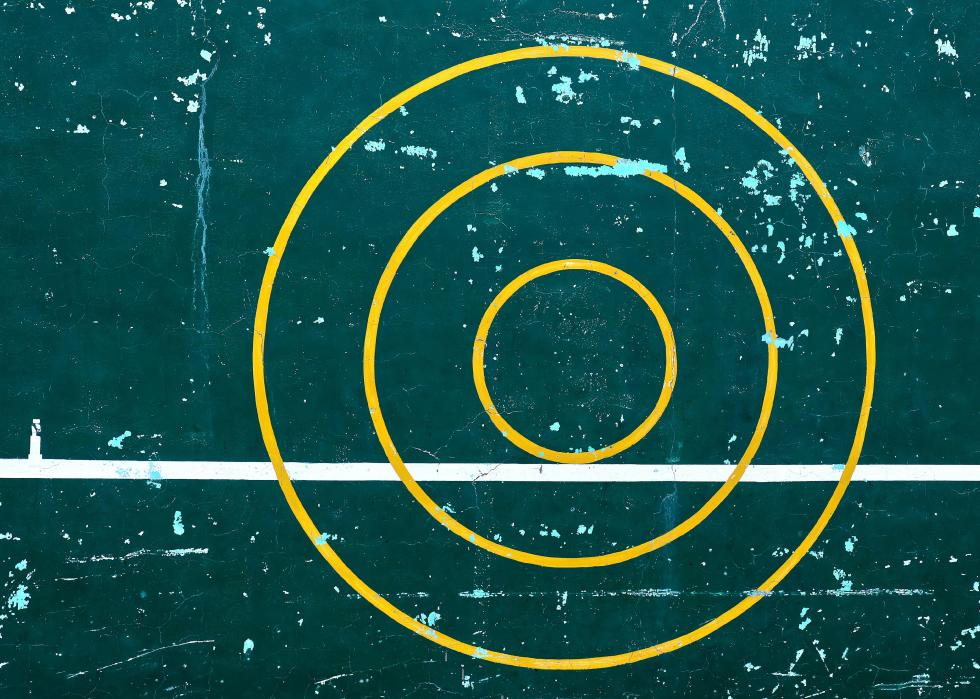19 / 50
jflin98 // Shutterstock

## Tangent

A tangent is a straight line that passes through a curve and has the slope of the curve at the point of intersection. The concept can also be applied to planes intersecting with curves.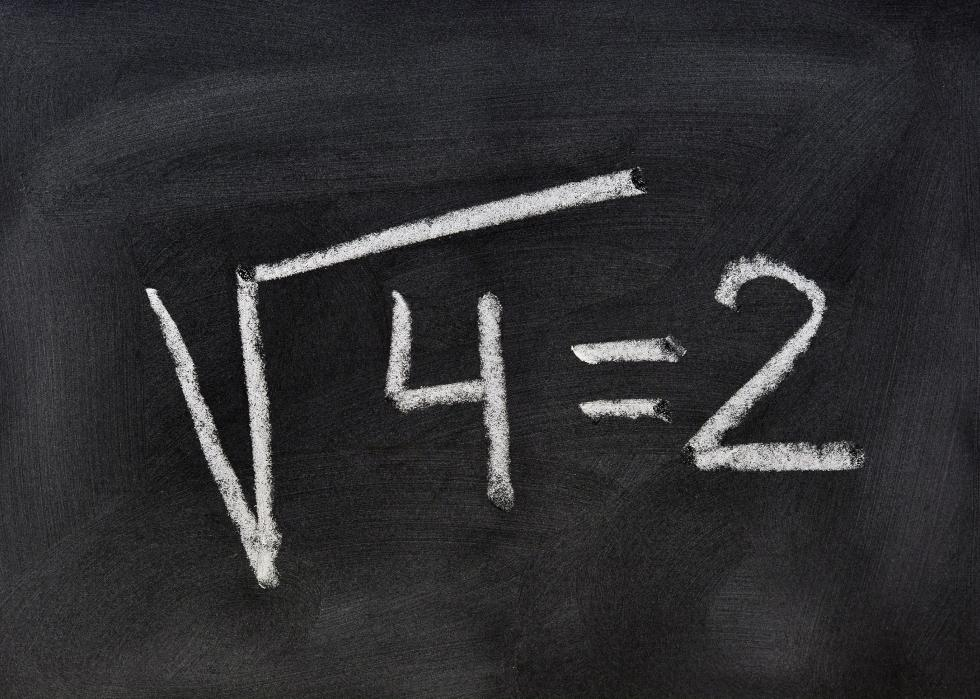20 / 50
Kuki Ladron de Guevara // Shutterstock

## Root

Roots are numbers that, when multiplied by themselves a given number of times, equal a different number. The square root of 9 is 3. The cube root of 64 is 4.

You may also like: Most popular dog breed the year you were born21 / 50

## Mean

Mean is a mathematical term for the concept commonly defined as "average." The mean is determined by adding all the numbers in a list and then dividing the sum by the number of numbers in the list.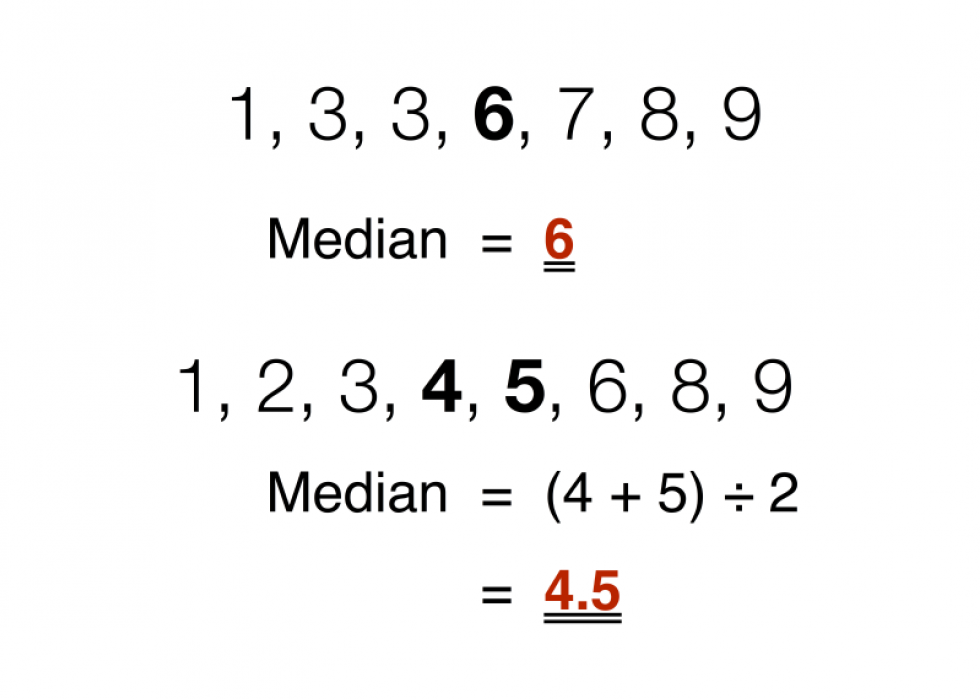22 / 50
Blythwood // Wikimedia Commons

## Median

Median numbers are like mean or average numbers, but the concept is used to determine the number that falls in the middle of a list of numbers. In the list 0, 1, 2, 4, 5, for example, the mean is 2.4. The median is 2.23 / 50
Nejron Photo // Shutterstock

## Odds

Every gambler in the world understands the concept of odds, even if they don't know the mathematical breakdown of the term. Odds are the probability that an event will occur—the number of times it's expected to happen in a set number of trials—divided by the probability that it will not occur.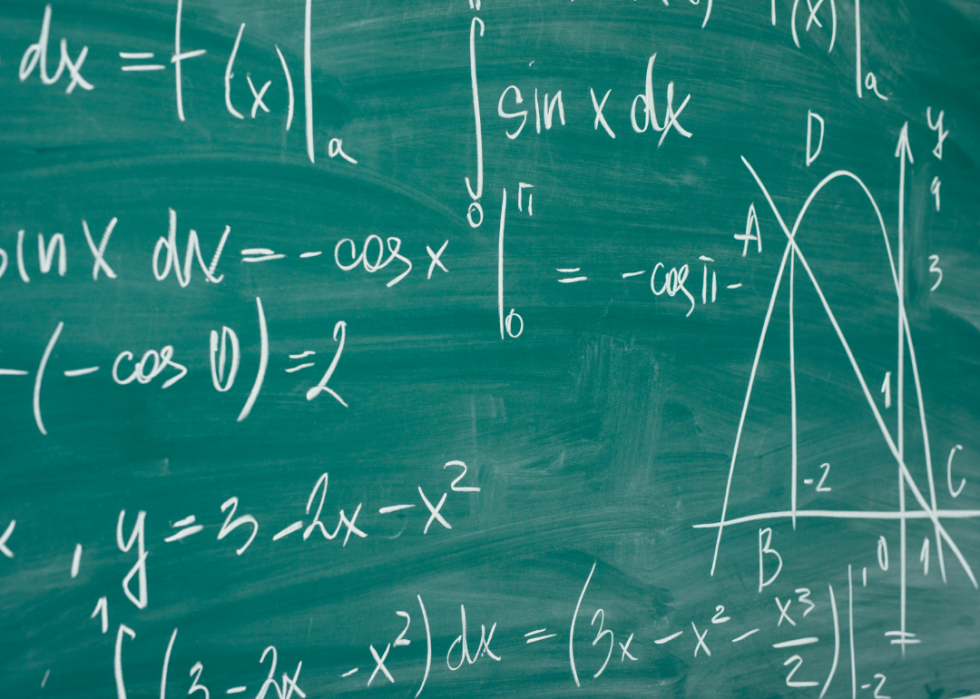24 / 50
Canva

## Abstract algebra

Standard algebra deals with regular number systems. Abstract algebra deals with abstract structures, the most important of which are fields, rings, and groups. Branches of abstract algebra include homological algebra, representation theory, and commutative algebra.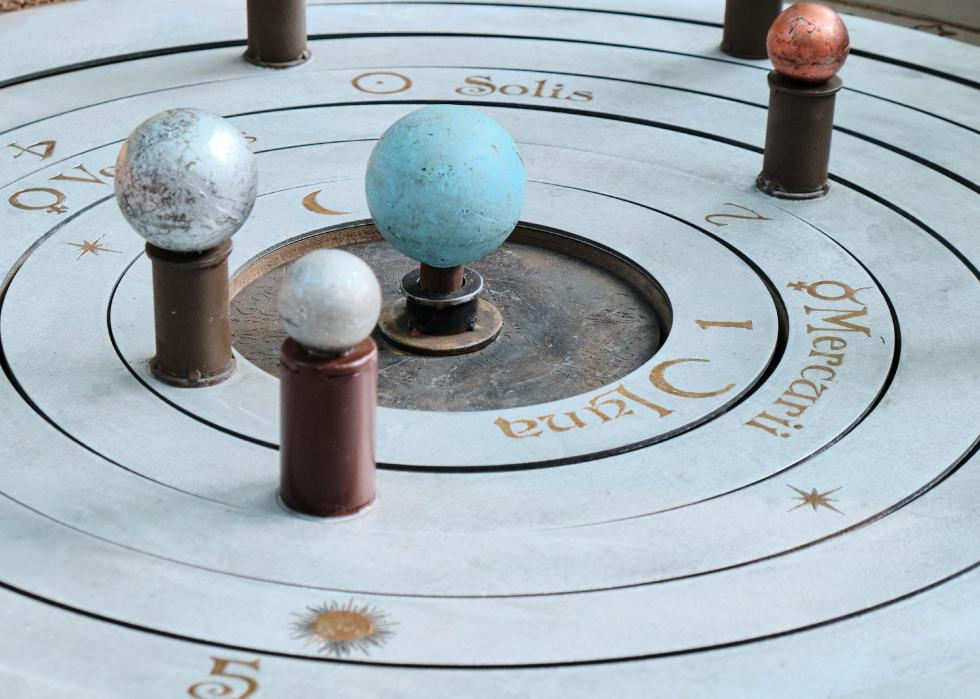25 / 50
Zhuravlev Andrey // Shutterstock

## Linear algebra

Despite the name, linear algebra is not actually algebra in the technical sense. It is involved in nearly all areas of mathematics and is fundamental to geometry. It allows for rotations while defining standard geometric shapes like planes and lines.

You may also like: States sending the most young people to the military26 / 50
fdecomite // Flickr

## Topology

Topology is the study of geometric objects whose shapes are preserved, even when twisted, stretched, or otherwise deformed. Möbius strips are common subjects of topology, as are trefoil knots.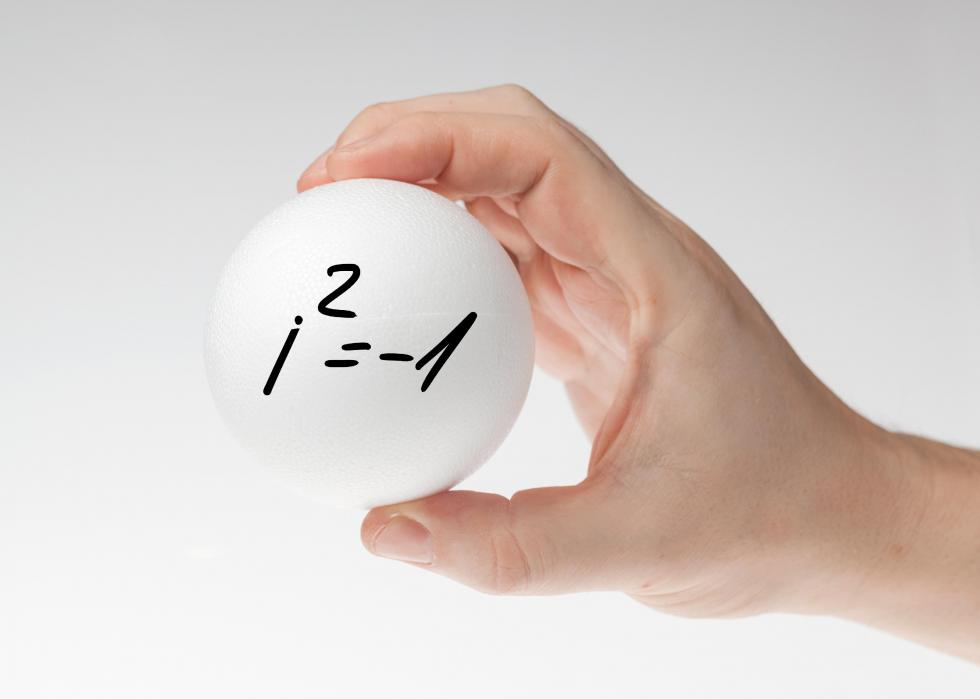27 / 50
Ivica Drusany // Shutterstock

## Complex number

Complex numbers are the combination of real numbers and "imaginary" numbers. Virtually every number is a real number since real numbers represent the value of a continuous quantity. Imaginary numbers are unusual numbers that give a negative result when squared.28 / 50
Travis Wolfe // Shutterstock

## Game theory

Game theory is a mathematical formulation used by biologists, social scientists, and economists. It's a complex system that is used to analyze and predict logical decision making in humans, animals, and computers.29 / 50
Patrick Foto // Shutterstock

## Chaos theory

Chaos theory studies how tiny changes in parts of a system can create enormous differences in the overall behavior of the larger system. The most famous representation is the so-called butterfly effect, which imagines that the flapping of a butterfly's wings on one continent could trigger a chain of events that lead to a tornado on another continent.30 / 50
Anton Novak // Shutterstock

## Taylor series

A Taylor series represents an expansion of a function into a sum of terms that is infinite. The exponents of its variables increase with no end.

You may also like: American history from the year you were born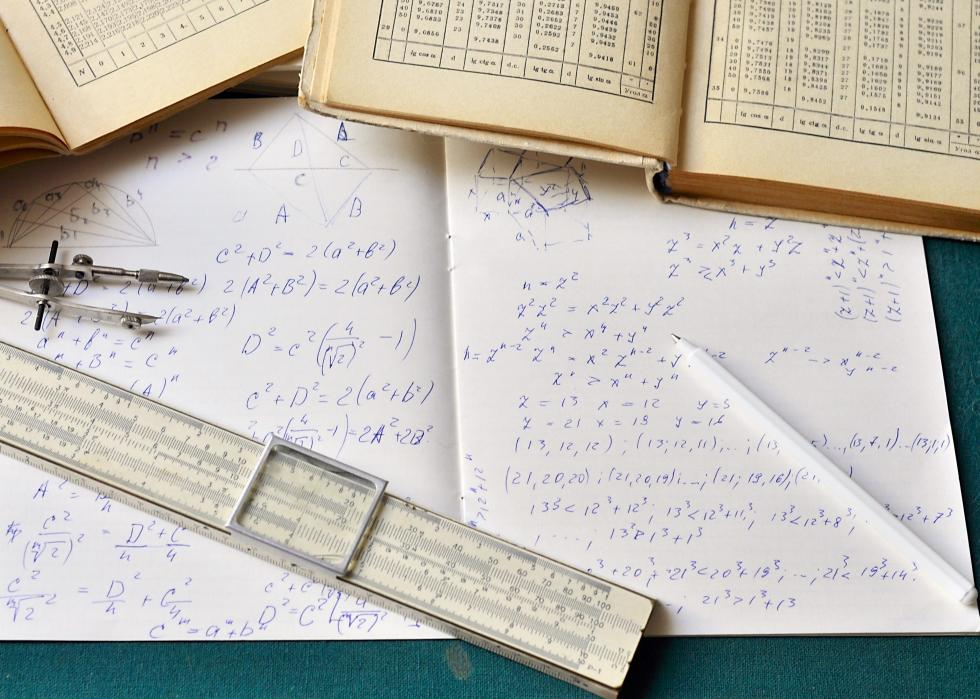31 / 50
sokolenok // Shutterstock

## Theorem

Theorems are statements that are not self-evident, but that can be proved and deduced by relying on previously accepted truths. This can include previously proved theorems.32 / 50
wutzkohphoto // Shutterstock

## Postulate

Unlike theorems, postulates are statements that are assumed to be true, even though there isn't any proof. They sometimes serve as starting points for proving other statements, and other times they are used to explain terms that are undefined.33 / 50
Chepko Danil Vitalevich // Shutterstock

## Divergence

Divergence is a concept in vector calculus. It, along with curl, is one of the vector operators that show themselves when mathematicians view vector fields like the flow of gas or fluid.34 / 50
SAWITRE INTAYAM // Shutterstock

## Convergence

The concept of convergence has applications in logic, computer science, and math. It deals with infinite functions coming closer to a limit as a variable—or an argument when dealing with logic—that increases or decreases along with the number of terms in the series.35 / 50
f11photo // Shutterstock

## Arc

An arc is any portion of the circumference of a circle. It's found by measuring the curved portion of a circle that exists between any two radius endpoints reaching from the center of the circle to the circle's enclosing circumference.

You may also like: Most popular baby names in the 21st century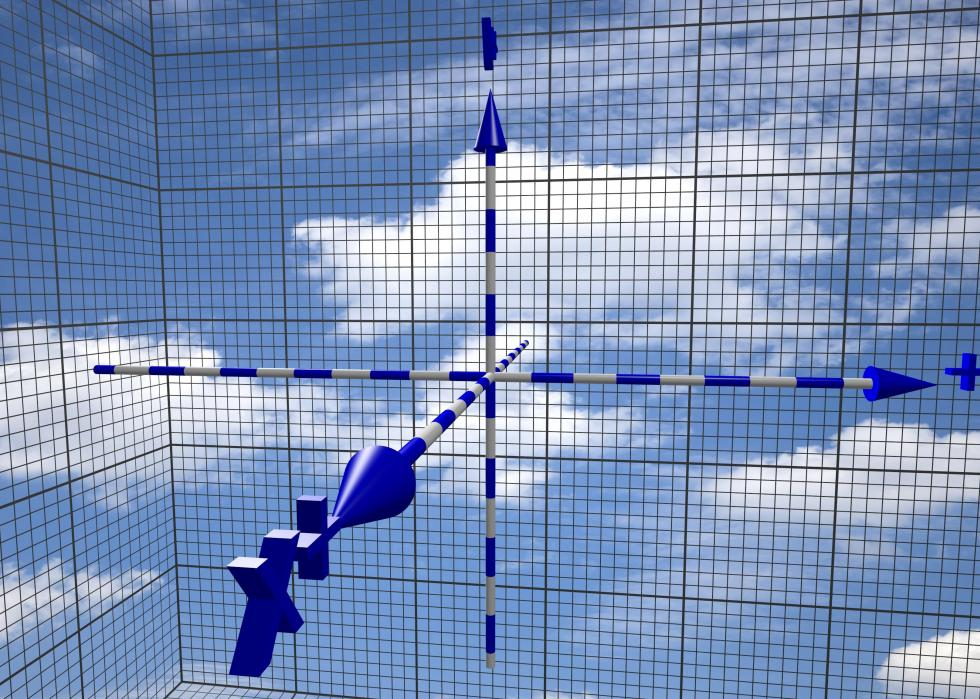36 / 50
sbellott // Shutterstock

## Cartesian coordinates

Cartesian coordinates show the location of a point in relation to a fixed point of reference called an origin. Mathematicians use them to chart both how far up and how far along points are on graphs.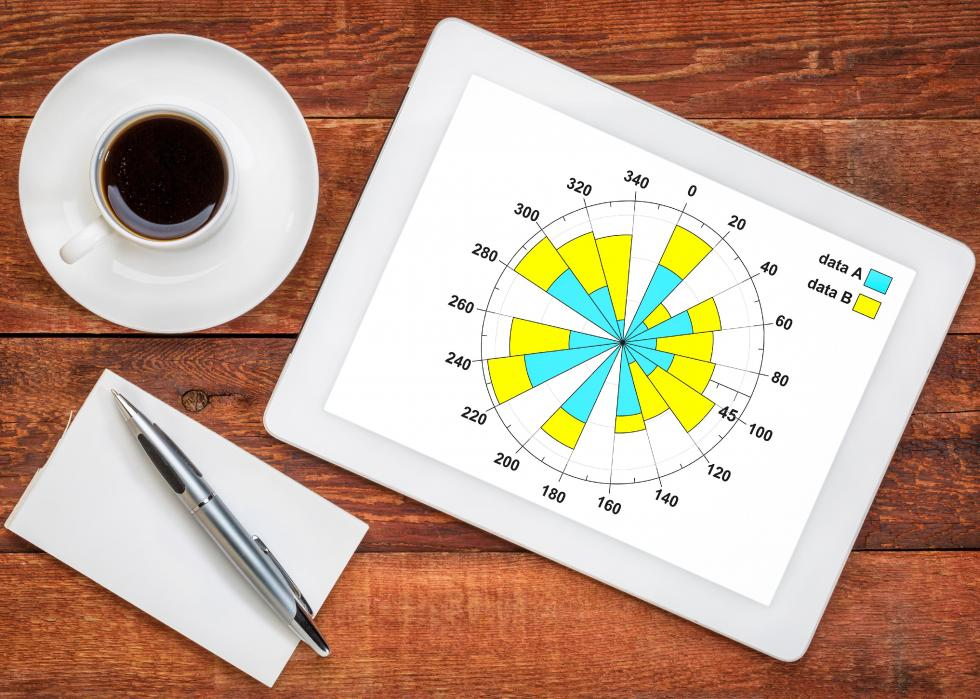37 / 50
marekuliasz // Shutterstock

## Polar coordinates

Like Cartesian coordinates, polar coordinates also help mathematicians identify points on graphs. Here, however, they're used to show how far away and at what angles the points are located.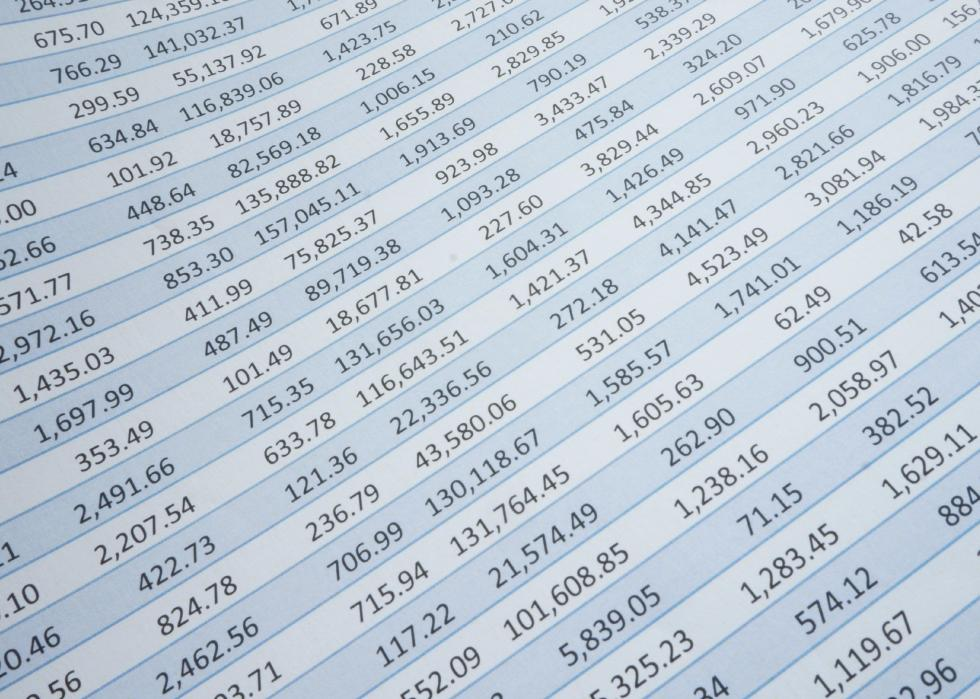38 / 50
Aaban // Shutterstock

## Series

A series is the sum of a given number of terms in a sequence. For example, in the sequence "3, 5, 7, 9," 8 (3+5) could be a series, as could 16 (9+7) and 15 (3+5+7).39 / 50
Allusioni // Shutterstock

## Vector

Vectors show the magnitude and direction of lines. Direction indicates which way a line is moving. Magnitude represents the length of the line.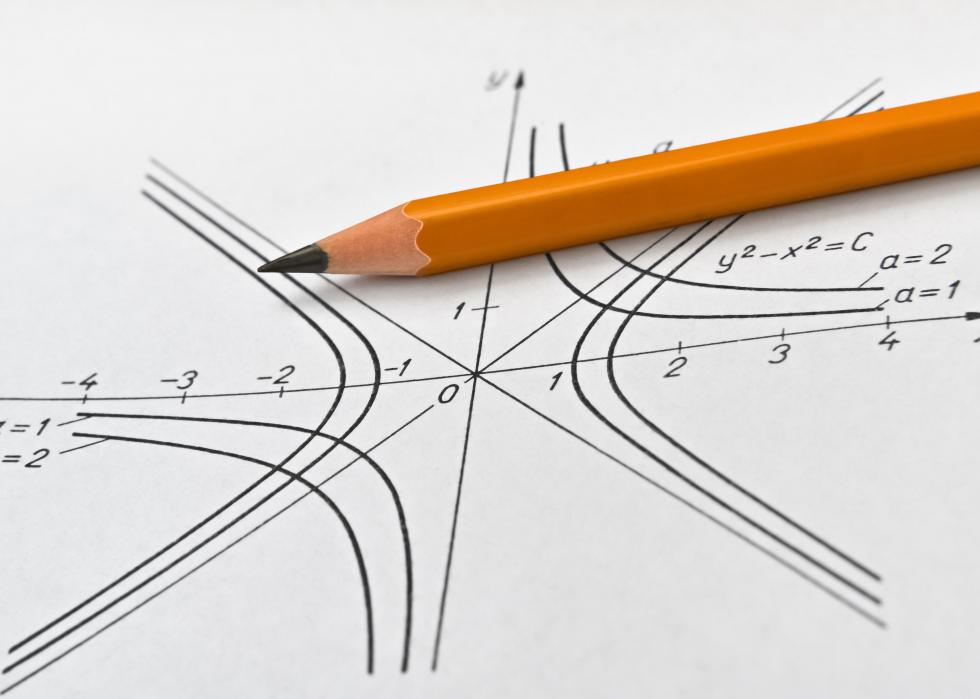40 / 50
benjaminec // Shutterstock

## Asymptote

In math, asymptotes can be vertical, horizontal, or slanted lines that graphs can approach, but can never touch. It's the mathematical equivalent of walking toward a fixed object at a pace that gets gradually slower and slower. Although the object gets closer and closer, the person doing the walking will never actually reach it.

You may also like: 50 endangered species that only live in the Amazon Rainforest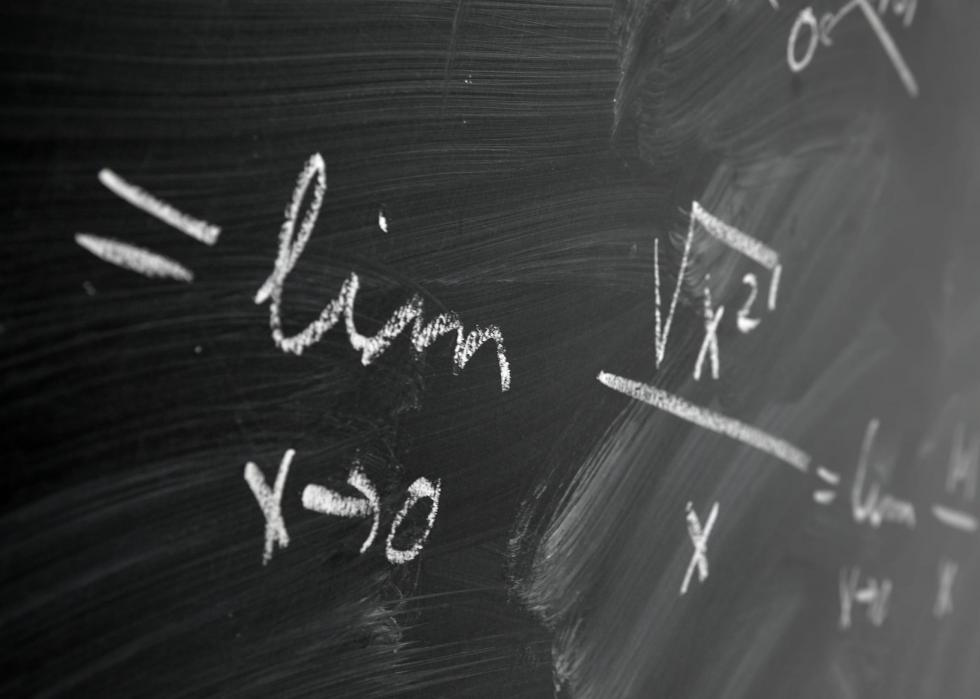41 / 50
Peter Gudella // Shutterstock

## Limits

Limits are critical components of calculus. They represent a realization of what the value or answer to a problem will be without actually being able to get to the solution.42 / 50
Efetova Anna // Shutterstock

## Rates of change

Rates of change represent increases or decreases in value between two points of data over time. Rates of change can be both positive and negative. Where there is no change, it's called a zero rate of change.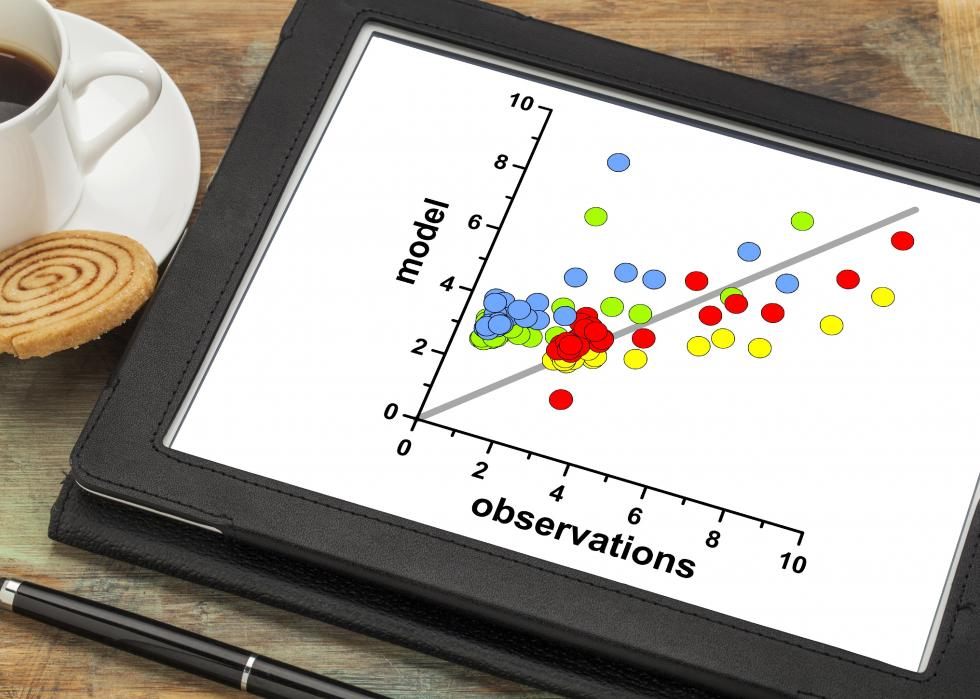43 / 50
marekuliasz // Shutterstock

## Correlation

Mathematicians use the concept of correlation to represent how strongly two sets of data are linked together. When two values increase together, the correlation is positive. When one decreases and the other increases, the correlation is negative.44 / 50
totojang1977 // Shutterstock

## Identity

Identities are equations that are true for every possible value and variable in a problem. Although it's technically appropriate to use three stacked horizontal lines to represent equations that are identities, many mathematicians simply use a traditional equal sign.45 / 50
NavinTar // Shutterstock

## Induction

Induction is a simple, two-step method that allows mathematicians to prove things. The first step is to show that something is true for the first occurrence. Step two is to show that if the first one is true, the next one is, too.

You may also like: Most popular baby names for boys the year you were born

[Pictured: Induction cooktop.]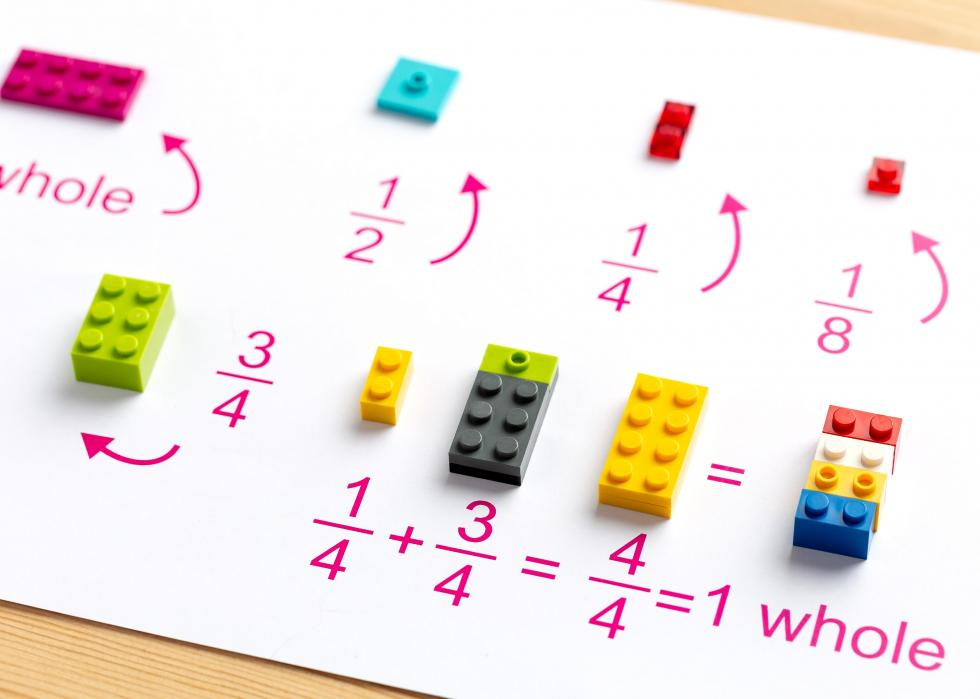46 / 50
Tatyana Abramovich // Shutterstock

## Integers

Whole numbers are standard, familiar, non-fraction numbers, including zero, like 0, 1, 2, 3, 4, and 5. Integers are also whole numbers, but they can also include non-fractional negative numbers.47 / 50
graphicINmotion // Shutterstock

## Matrix

A matrix is a group of numbers arranged in rows and columns in a rectangular pattern. More than one matrix are matrices.48 / 50
6377724229 // Shutterstock

## Permutations and combinations

Combinations are groups of numbers where the order isn't important, and permutations are groups of numbers where the order is predetermined. This is the opposite of common language—kids in school should refer to their locker permutations, not their locker combinations.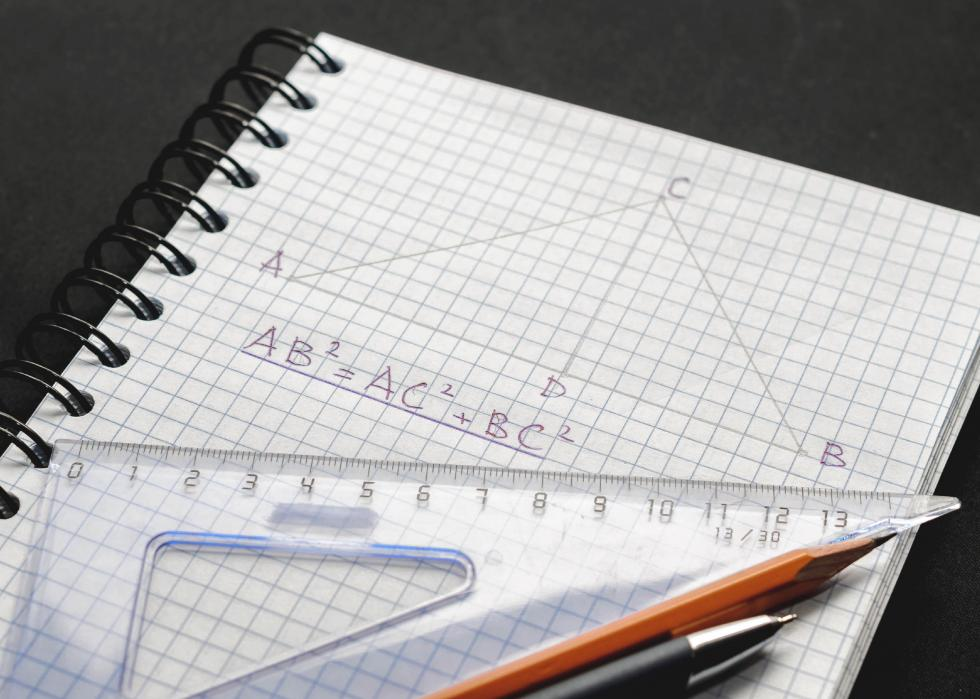49 / 50
Maksim Gusarov // Shutterstock

## Pythagorean theorem

The Pythagorean theorem is one of the most famous concepts in math. It tells mathematicians about the relationship between the sides of a right triangle. According to the Pythagorean theorem, that relationship is always a² + b² = c².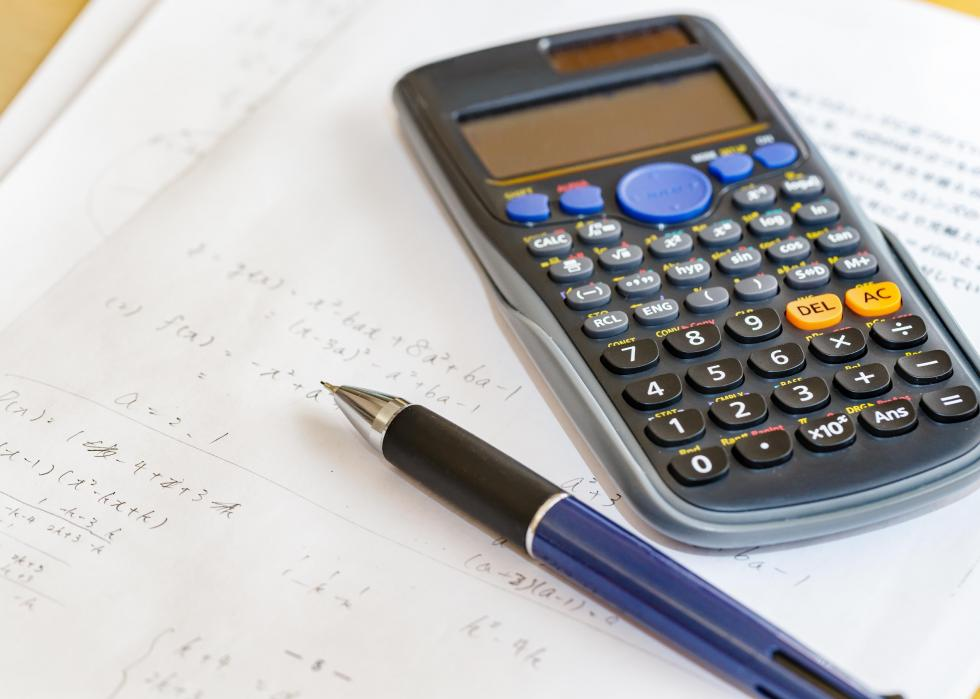50 / 50
taka1022 // Shutterstock

## Reciprocal

To find a number's reciprocal, mathematicians divide one by that number. For example, the reciprocal of 4 is 1/4.

You may also like: States doing the most for a clean energy future

## 50 best movies of the '60s## Top 100 country songs of all time## 50 best colleges on the East Coast## 50 best crime TV shows of all time